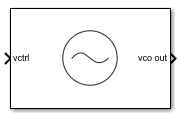# VCO

Model voltage controlled oscillator

• Library:
• Mixed-Signal Blockset / PLL / Building Blocks

•## Description

VCO or voltage controlled oscillator is a voltage to frequency converter. It produces an output square wave signal whose frequency is controlled by the voltage at the input vctrl port. The frequency of the output signal, F is determined either by:

$F=\left({K}_{VCO}·{V}_{ctrl}\right)+{F}_{o}$

where:

• Kvco = voltage sensitivity (in Hz/V)

• Vctrl = control voltage (in V)

• Fo= free running frequency (in Hz)

or from linear interpolation using the mapping:

$F=\text{interp}\left({F}_{out}\left({V}_{cntl}\right)\right)$

where:

• Vctnl = vector of control voltages (in V)

• Fout= vector of corresponding output frequencies (in Hz)

## Ports

### Input

expand all

VCO control voltage used to control the output frequency of the VCO. In a phase-locked loop (PLL) system, vctrl is the output of the Loop Filter that contains the phase error information.

Data Types: `double`

### Output

expand all

Output square wave signal of VCO. In a PLL system, vco out is the output clock generated by the PLL. It is also fed back to the PFD block through a clock divider to complete the control loop.

Data Types: `double`

## Parameters

expand all

### Parameters

Define how VCO output frequency is specified:

• Select `Voltage sensitivity` to specify output frequency from Voltage sensitivity (Hz/V) and Free running frequency (Hz).

• Select ```Output frequency vs. control voltage``` to interpolate output frequency from Control voltage (V) vector versus Output frequency (Hz) vector.

#### Programmatic Use

 Block parameter: `SpecifyUsing` Type: character vector Values: `Voltage sensitivity` | ```Output frequency vs. control voltage``` Default: `Voltage sensitivity`

Measure of change in output frequency for input voltage change, specified as a positive real scalar with units in Hz/V. This parameter is also reported as VCO voltage sensitivity in the Loop Filter tab and is used to automatically calculate the filter component values of the loop filter.

#### Dependencies

To enable this parameter, select ```Voltage sensitivity``` in Specify using in the Parameters tab.

#### Programmatic Use

 Block parameter: `Kvco` Type: character vector Values: positive real scalar Default: `100e6`

Data Types: `double`

Frequency of the VCO without any control voltage input (`0` V), or the quiescent frequency, specified as a positive real scalar in Hz.

#### Dependencies

To enable this parameter, select ```Voltage sensitivity``` in Specify using in the Parameters tab.

#### Programmatic Use

 Block parameter: `Fo` Type: character vector Values: positive real scalar Default: `2.5e9`

Data Types: `double`

Control voltage values of the VCO, specified as a real valued vector in volts.

#### Dependencies

To enable this parameter, select ```Output frequency vs. control voltage``` in Specify using in the Parameters tab.

#### Programmatic Use

 Block parameter: `ControlVoltage` Type: character vector Values: real valued vector Default: `[-5 0 5]`

Data Types: `double`

Output frequency of the values of the VCO, corresponding to the Control voltage (V) vector, specified in Hz.

#### Dependencies

To enable this parameter, select ```Output frequency vs. control voltage``` in Specify using in the Parameters tab.

#### Programmatic Use

 Block parameter: `OutputFrequency` Type: character vector Values: positive real valued vector Default: `[2e9 2.5e9 3e9]`

Data Types: `double`

Maximum amplitude of the VCO output voltage, specified as a positive real scalar.

#### Programmatic Use

 Block parameter: `Amplitude` Type: character vector Values: positive real scalar Default: `1`

Data Types: `double`

Select to enable increased buffer size during simulation. This increases the buffer size of the Variable Pulse Delay block inside the VCO block. By default, this option is deselected.

Number of samples of the input buffering available during simulation, specified as a positive integer scalar. This sets the buffer size of the Variable Pulse Delay block inside the VCO block.

Selecting different simulation solver or sampling strategies can change the number of input samples needed to produce an accurate output sample. Set the Buffer size to a large enough value so that the input buffer contains all the input samples required.

#### Dependencies

To enable this parameter, select Enable increased buffer size.

#### Programmatic Use

 Block parameter: `NBuffer` Type: character vector Values: positive integer scalar Default: `10`

Data Types: `double`

### Impairments

Select to introduce phase noise as a function of frequency to the VCO. By default, this option is selected.

The frequency offsets of phase noise from the carrier frequency specified as a positive real valued vector in Hz.

#### Dependencies

To enable this parameter, select Add phase noise in the Impairments tab.

#### Programmatic Use

 Block parameter: `Foffset` Type: character vector Values: positive real valued vector Default: `[30e3 100e3 1e6 3e6 10e6]`

Data Types: `double`

The phase noise power in a 1 Hz bandwidth centered at the specified frequency offsets relative to the carrier specified as a negative real valued vector in dBc/Hz. The elements of Phase noise level corresponds to relative elements in the Phase noise frequency offset.

#### Dependencies

To enable this parameter, select Add phase noise in the Impairments tab.

#### Programmatic Use

 Block parameter: `PhaseNoise` Type: character vector Values: negative real valued vector Default: `[-56 -106 -132 -143 -152]`

Data Types: `double`

expand all

 Banerjee, Dean. PLL Performance, Simulation and Design. Indianapolis, IN: Dog Ear Publishing, 2006.

## SupportGet trial now